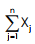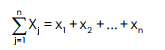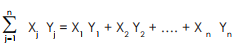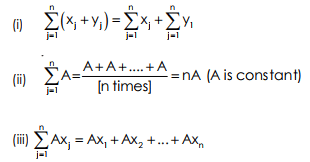# Introduction - Measures of Central Tendency, Business Mathematics & Statistics Notes | Study Business Mathematics and Statistics - B Com

## B Com: Introduction - Measures of Central Tendency, Business Mathematics & Statistics Notes | Study Business Mathematics and Statistics - B Com

The document Introduction - Measures of Central Tendency, Business Mathematics & Statistics Notes | Study Business Mathematics and Statistics - B Com is a part of the B Com Course Business Mathematics and Statistics.
All you need of B Com at this link: B Com

MEASURES OF CENTRAL TENDENCY OR AVERAGE INTRODUCTION :
A given raw statistical data can be condensed to a large extent by the methods of classification and tabulation. But this is not enough. For interpreting a given data we are to depend on some mathematical measures. Such a type of measure is the measure of Central Tendency. By the term of ‘Central Tendency of a given statistical data’ we mean that central value of the data about which the observations are concentrated . A central value which ‘enables us to comprehend in a single effort the significance of the whole is known as Statistical Average or simply average. The three common measures of Central Tendency are :
(i) Mean
(ii) Median
(iii) Mode

The most common and useful measure is the mean. As we proceed, we shall discuss the methods of computation of the various measures. In all such discussions, we need some very useful notations, which we propose to explain before proceeding any further.
(i) Index or Subscript Notation : Let X be a variable assuming n values x1 , x2 , …..x3 , We use the symbol x j (read “x sub j”) to denote any of the above mentioned n numbers. The letter j, which can stand for any of the numbers x1 , x2 , …..x n is called a subscript notation of index. Obviously, any letter other than j, as I, k, p, q and s could be used.’
(ii) Summation Notation :

The symbolis used to denote the sum xj ’s from j = 1 to j = n. By definition.Example 1 :The document Introduction - Measures of Central Tendency, Business Mathematics & Statistics Notes | Study Business Mathematics and Statistics - B Com is a part of the B Com Course Business Mathematics and Statistics.
All you need of B Com at this link: B ComUse Code STAYHOME200 and get INR 200 additional OFF

122 videos|142 docs

Track your progress, build streaks, highlight & save important lessons and more!

,

,

,

,

,

,

,

,

,

,

,

,

,

,

,

,

,

,

,

,

,

,

,

,

;